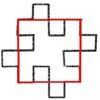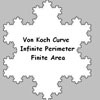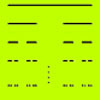#### You may also like### Squareflake

A finite area inside and infinite skin! You can paint the interior of this fractal with a small tin of paint but you could never get enough paint to paint the edge.### Von Koch Curve

Make a poster using equilateral triangles with sides 27, 9, 3 and 1 units assembled as stage 3 of the Von Koch fractal. Investigate areas & lengths when you repeat a process infinitely often.### How Many Elements Are There in the Cantor Set?

This article gives a proof of the uncountability of the Cantor set.

# Sierpinski Triangle

##### Age 16 to 18 Challenge Level:

Thank you Jeremy from Drexel University, Philadelphia, USA and Andrei, from Tudor Vianu National College, Bucharest, Romania for two more excellent solutions.

To solve this problem, first I made a table, and I filled it with the properties of the figures in the problem.

 Stage Number of red triangles Area of red triangles Number of white triangles Area of white triangles $0$ $1$ $1$ $0$ $1-1$ $1$ $3$ $3\over 4$ $1$ $1-{3\over 4}$ $2$ $3 \times3$ ${3\over 4}\times {3\over 4}$ $1+3$ $1-\left({3\over 4}\right)^2$

From the analysis of the passage from one stage to the next, I made some observations:

• The number of red triangles at each stage is multiplied by three to give the number of red triangles at the next stage.
• The total area of the red triangles at each stage is multiplied by 3/4 to give the total area of the red triangles at the next stage.
• For each red triangle at stage $n$ one additional white triangle appears at stage $n+1$. Equivalently the number of white triangles added at each stage is three times the number of white triangles added at the previous stage.
• The total area of all the white triangles is [1 - (total area of red triangles)].
In the table below, I summarised the results obtained from these observations, and I have also calculated the limits for $n \to \infty$.[Jeremy gave a proof by induction for each of these formulae]

 Stage Number ofred triangles Area of red triangles Number of white triangles Area of white triangles $n$ $3^n$ $\left(\frac{3}{4}\right)^n$ $1 + 3 +3^2 ... +3^{n-1}= {3^n -1\over 2}$ $1-\left(\frac{3}{4}\right)^n$ $n \to \infty$ $\infty$ $0$ $\infty$ $1$

The total area of the white triangles (that is the triangles removed) is given by the series: $$\left({1\over 4}\right) + 3\left({1\over 4}\right)^2 + 3^2\left({1\over 4}\right)^3 +... 3^{n-1}\left({1\over4}\right)^n = 1 - \left({3\over 4}\right)^n$$ and this is as expected from the calculation of the total area of the red triangles.

In order to calculate the dimension $d$ from the formula $n=m^d$ , where $n$ is the number of self similar pieces and $m$ is the magnification factor, I see that for this problem $n=3$ and $m = 2$. So, $3 = 2^d$ which gives $\log 3 = \log\left(2^d\right)$ and hence $$d = {\log 3\over \log 2} =\log_2 3\approx 1.58$$ I see that the dimension $d$ is between $1$ and $2$, as expected.